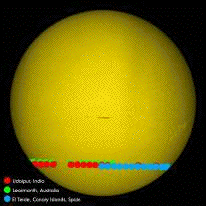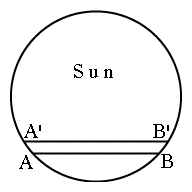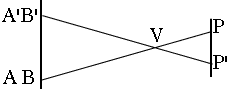# #12c   The 2004 Transit of Venus --

## Halley's Method of Deriving the AU

### (First of 3 linked optional sections)

For the background to this calculation, see "The Scale of the Solar System".Index10a. Scale of Solar Sys.11. Graphs & Ellipses11a. Ellipses
and First Law12. Second Law12a. More on 2nd Law12b. Orbital Motion12c. Venus transit (1)12d. Venus transit (2)12e. Venus transit (3)

Newtonian Mechanics13. Free Fall14. VectorsFig. 1   The 2004 Venus transit observed from 3 locations by the GONG collaboration

Kepler's third law allows one to evaluate the dimensions of the solar system in relative units, e.g. in "astronomical units" (AUs), where 1 AU is the mean Sun-Earth distance. However, to express the AU in kilometers or in miles seems to require some sort of parallax--some difference in the observed position of some object in the Sun's system, when seen from two separated points, the distance between which (in kilometers or miles) is known. The problem is, planetary objects are so enormously distant, that the shift in their apparent position, when viewed from two separated locations on Earth, is tiny small.

Edmond Halley suggested using the transit of Venus across the Sun's disk, when it appears as a dark round spot--or to quote one observer of the 2004 transit, "like a blueberry in front of an orange." This is a relatively rare event. Transits occur in pairs more than a century apart: none took place during the 1900s, though one occurred on June 8, 2004, with another in 2012. Information on the 2004 transit (for which this page was originally prepared) is found here.Suppose some observer at point P on Earth sees Venus cross the Sun's disk from A to B (Figure 2 above--two figures, front and side). The motion of Venus across the Sun's disk is due to the combined orbital motions of the two planets;   by Kepler's laws, the closer a planet is to the Sun, the faster it moves, so Venus moves faster than Earth, and during a transit essentially overtakes it.     The orbital plane of Venus is quite close to the one of Earth, usually known as the plane of the ecliptic (close, but not identical--if it were, a transit would occur every time Venus overtakes Earth). Therefore the line AB is nearly parallel to the line marking the ecliptic on the celestial sphere, the line along which the plane of the ecliptic intersects our view of the sky.     Viewed from a different point P', Venus moves along A'B', essentially parallel to AB. That different point is preferably on the opposite side of the equator, giving them different latitudes. If we then measure the distance D between AB and A'B', we can in principle apply some simple trigonometry to the parallax and derive the distance PV between P and the position V of Venus.     If only astronomy were so easy! Actually, the lines AB and A'B' are very close to each other. The figure on top of the page contains images of Venus in front of the Sun, obtained on June 8, 2004, from 3 locations, with each set of data marked in a different color. It is evident the paths are very close, less than a Venus radius apart. Measuring each separately and deriving from that their separation D is not likely to give a very accurate result.     What Halley noted was that equivalent information can be obtained by timing the passage of Venus from A to B and from A' to B'. Because the edge ("limb") of the Sun curves, the length AB differs from that of A'B' (we say "length" for convenience--actually AB, A'B' and D are all visual angles). The apparent velocity of the motion of Venus across the Sun is almost the same when viewed from any location on Earth, so by obtaining the difference in time between the crossing of AB and of A'B', the distance D can also be deduced. This is much more sensitive because the duration of the transit is hours, while the difference in transit time may amount to minutes. Such durations can be (in principle) measured quite accurately.     These sections outline a simplified procedure for deriving the astronomical unit from the transit times on June 8, 2004, using predicted durations of the transits. The astronomers who calculated those durations (obtained from the web) here--used of course the well-established value of the astronomical unit, making this just an exercise. If this had been a "real" calculation, observed values would have been used. This will be a somewhat crude calculation, using simplifying assumptions and neglecting corrections which actual determinations may need.     Since we are free to select observing stations, we choose two at almost the same longitude and at equal latitudes north and south of the equator. They are both in Africa (latitude and longitude within about half a degree)

 Cairo Lat. 30 N Long. 32 E Durban Lat. 30 S Long 31 E
 This symmetry of positions simplifies the analysis. As noted above, the motion of Venus across the face of the Sun is almost entirely due to the orbital motions of Earth and Venus. With Earth orbiting at 30 km/s, one may think that the velocity contributed by the Earth's rotation can be neglected, since it typically amounts to only a few 100 meters/second. However, that velocity moves the observer during the entire duration of the transit, amounting to more than 5 hours. During that time some observers may be displaced by as much as one Earth radius, and the difference in displacement between observers at different latitudes may reach several thousand kilometers.     On the other hand, the information from which the astronomical unit is derived is contained in a time difference ΔT of only about 5 minutes, during which the Earth moves by about 300 sec × 30 km/s = 9000 km An added displacement of a few 1000 km could seriously degrade the result, and may require an added correction. To simplify the calculation here, we sidestep this problem by selecting two sites equally distant from the equator, and therefore with equal rotational velocities. Choosing nearly the same longitude also evens out velocities with respect to Venus and the Sun. Thus both transit times are affected almost equally, and ΔT will be used without the extra correction.     This web page is linked to two others.     The next page gives the derivation of D. The calculation requires some of the trigonometry developed in the "Math Refresher" and in particular the formulas for the sine and the cosine of sums of angles, derived in section M-11.     The page following this derives an approximate value of the astronomical unit. Because of the approximations used, the result is off by several percent, but it requires no mathematical tools beyond the ones covered here. You will also encounter some of the complications with which astronomers must contend. Questions from Users: *** Rotation of Venus

Next linked section:       #12d   Derivation of the displacement angle D

Author and Curator:   Dr. David P. Stern
Mail to Dr.Stern:   stargaze("at" symbol)phy6.org .

Last updated: 10 October 2016